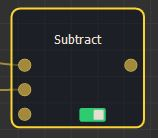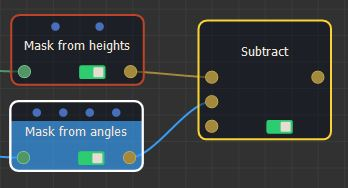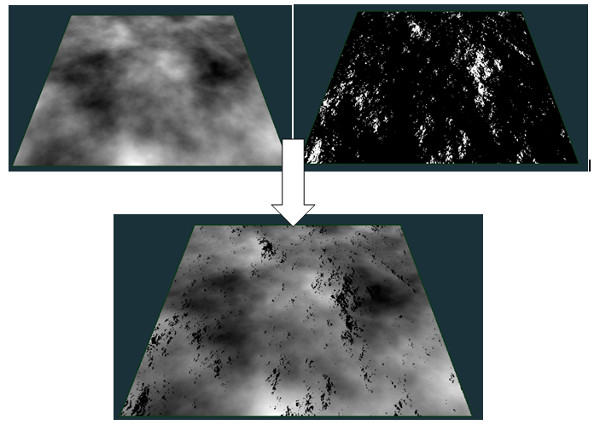### Adding a mask Subtract node

This node subtracts one mask from another mask. At each vertex, the value of the output mask is the difference of the values of both input masks.

To add a node, right-click in the Graph Editor and select Create Node  > Mask Composition > Difference.This node has no properties.

### Linking to input masks

Link the Subtract node to two input nodes.The minimum value for a mask is 0. At a given vertex, if the difference between the values of both masks is less than 0, the output mask will have a value 0 at this vertex and not a value less than 0. If both input masks do not have the same size or resolution, the size and resolution of the output mask is determined by the size and resolution of the first mask, and the second mask is enlarged or decreased so that its size corresponds exactly to the size of the output mask, before being subtracted from the first mask.

For example:Copyright © 2021 · All Rights Reserved · Wysilab